# Existence results for nonlocal boundary value problems of fractional differential equations and inclusions with strip conditions

## Abstract

This article studies a new class of nonlocal boundary value problems of nonlinear differential equations and inclusions of fractional order with strip conditions. We extend the idea of four-point nonlocal boundary conditions $\left(x\left(0\right)=\sigma x\left(\mu \right),x\left(1\right)=\eta x\left(v\right),\sigma ,\eta \in ℝ,\phantom{\rule{2.77695pt}{0ex}}0<\mu ,v<1\right)$ to nonlocal strip conditions of the form: $x\left(0\right)=\sigma {\int }_{\alpha }^{\beta }x\left(s\right)ds$, $x\left(1\right)=\eta {\int }_{\gamma }^{\delta }x\left(s\right)ds,\phantom{\rule{2.77695pt}{0ex}}0\phantom{\rule{2.77695pt}{0ex}}<\phantom{\rule{2.77695pt}{0ex}}\alpha \phantom{\rule{2.77695pt}{0ex}}<\beta \phantom{\rule{2.77695pt}{0ex}}<\gamma \phantom{\rule{2.77695pt}{0ex}}<\delta \phantom{\rule{2.77695pt}{0ex}}<\phantom{\rule{2.77695pt}{0ex}}1$. These strip conditions may be regarded as six-point boundary conditions. Some new existence and uniqueness results are obtained for this class of nonlocal problems by using standard fixed point theorems and Leray-Schauder degree theory. Some illustrative examples are also discussed.

MSC 2000: 26A33; 34A12; 34A40.

## 1 Introduction

The subject of fractional calculus has recently evolved as an interesting and popular field of research. A variety of results on initial and boundary value problems of fractional order can easily be found in the recent literature on the topic. These results involve the theoretical development as well as the methods of solution for the fractional-order problems. It is mainly due to the extensive application of fractional calculus in the mathematical modeling of physical, engineering, and biological phenomena. For some recent results on the topic, see  and the references therein.

In this article, we discuss the existence and uniqueness of solutions for a boundary value problem of nonlinear fractional differential equations and inclusions of order q (1, 2] with nonlocal strip conditions. As a first problem, we consider the following boundary value problem of fractional differential equations

$\left\{\begin{array}{c}\hfill {}^{c}{D}^{q}x\left(t\right)=f\left(t,x\left(t\right),\phantom{\rule{1em}{0ex}}0
(1.1)

where cDq denotes the Caputo fractional derivative of order q, $f\phantom{\rule{2.77695pt}{0ex}}:\phantom{\rule{2.77695pt}{0ex}}\left[0,1\right]×\phantom{\rule{0.3em}{0ex}}ℝ\to ℝ$ is a given continuous function and σ, η are appropriately chosen real numbers.

The boundary conditions in the problem (1.1) can be regarded as six-point nonlocal boundary conditions, which reduces to the typical integral boundary conditions in the limit α, γ → 0, β, δ → 1. Integral boundary conditions have various applications in applied fields such as blood flow problems, chemical engineering, thermoelasticity, underground water flow, population dynamics, etc. For a detailed description of the integral boundary conditions, we refer the reader to the articles [20, 21] and references therein. Regarding the application of the strip conditions of fixed size, we know that such conditions appear in the mathematical modeling of real world problems, for example, see [22, 23].

As a second problem, we study a two-strip boundary value problem of fractional differential inclusions given by

$\left\{\begin{array}{c}\hfill {}^{c}{D}^{q}x\left(t\right)\in F\left(t,x\left(t\right)\right),\phantom{\rule{1em}{0ex}}0
(1.2)

where $F\phantom{\rule{2.77695pt}{0ex}}:\phantom{\rule{2.77695pt}{0ex}}\left[0,1\right]×ℝ\to \mathcal{P}\left(ℝ\right)$ is a multivalued map, $\mathcal{P}\left(ℝ\right)$ is the family of all subsets of .

We establish existence results for the problem (1.2), when the right-hand side is convex as well as non-convex valued. The first result relies on the nonlinear alternative of Leray-Schauder type. In the second result, we shall combine the nonlinear alternative of Leray-Schauder type for single-valued maps with a selection theorem due to Bressan and Colombo for lower semicontinuous multivalued maps with nonempty closed and decomposable values, while in the third result, we shall use the fixed point theorem for contraction multivalued maps due to Covitz and Nadler.

The methods used are standard, however their exposition in the framework of problems (1.1) and (1.2) is new.

## 2 Linear problem

Let us recall some basic definitions of fractional calculus .

Definition 2.1 For at least n-times continuously differentiable function $g\phantom{\rule{2.77695pt}{0ex}}:\phantom{\rule{2.77695pt}{0ex}}\left[0,\infty \right)\to ℝ$, the Caputo derivative of fractional order q is defined as

${}^{c}{D}^{q}g\left(t\right)=\frac{1}{\mathrm{\Gamma }\left(n-q\right)}\underset{0}{\overset{t}{\int }}{\left(t-s\right)}^{n-q-1}{g}^{\left(n\right)}\left(s\right)ds,\phantom{\rule{2.77695pt}{0ex}}\phantom{\rule{1em}{0ex}}n-1

where [q] denotes the integer part of the real number q.

Definition 2.2 The Riemann-Liouville fractional integral of order q is defined as

${I}^{q}g\left(t\right)=\frac{1}{\mathrm{\Gamma }\left(q\right)}\underset{0}{\overset{t}{\int }}\frac{g\left(s\right)}{{\left(t-s\right)}^{1-q}}ds,\phantom{\rule{2.77695pt}{0ex}}\phantom{\rule{1em}{0ex}}q>0,$

provided the integral exists.

By a solution of (1.1), we mean a continuous function x(t) which satisfies the equation cDqx(t) = f (t, x(t)), 0 < t < 1, together with the boundary conditions of (1.1).

To define a fixed point problem associated with (1.1), we need the following lemma, which deals with the linear variant of problem (1.1).

Lemma 2.3 For a given $g\phantom{\rule{2.77695pt}{0ex}}\in \phantom{\rule{2.77695pt}{0ex}}C\left(\left[0,1\right],ℝ\right)$, the solution of the fractional differential equation

${}^{c}{D}^{q}x\left(t\right)=g\left(t\right),\phantom{\rule{2.77695pt}{0ex}}\phantom{\rule{1em}{0ex}}1
(2.1)

subject to the boundary conditions in (1.1) is given by

(2.2)

where

$\mathrm{\Delta }=\left[\frac{\eta }{2}\left({\delta }^{2}-{\gamma }^{2}\right)-1\right]\left[\sigma \left(\beta -\alpha \right)-1\right]-\left[\frac{\sigma }{2}\left({\beta }^{2}-{\alpha }^{2}\right)\right]\left[\eta \left(\delta -\gamma \right)-1\right]\ne 0.$

Proof. It is well known that the solution of (2.1) can be written as 

$x\left(t\right)={I}^{q}g\left(t\right)-{c}_{0}-{c}_{1}t=\underset{0}{\overset{t}{\int }}\frac{{\left(t-s\right)}^{q-1}}{\mathrm{\Gamma }\left(q\right)}g\left(s\right)ds-{c}_{0}-{c}_{1}t.$
(2.3)

where ${c}_{0},{c}_{1}\in ℝ$ are constants. Applying the boundary conditions given in (1.1), we find that

$\begin{array}{ll}\hfill \left(\sigma \left(\beta -\alpha \right)-1\right){c}_{0}+\frac{\sigma }{2}\left({\beta }^{2}-{\alpha }^{2}\right){c}_{1}& =\sigma \underset{\alpha }{\overset{\beta }{\int }}\left(\underset{0}{\overset{s}{\int }}\frac{{\left(s-m\right)}^{q-1}}{\mathrm{\Gamma }\left(q\right)}g\left(m\right)dm\right)ds,\phantom{\rule{2em}{0ex}}\\ \hfill \left(\eta \left(\delta -\gamma \right)-1\right){c}_{0}+\left(\frac{\eta }{2}\left({\delta }^{2}-{\gamma }^{2}\right)-1\right){c}_{1}& =\eta \underset{\gamma }{\overset{\delta }{\int }}\left(\underset{0}{\overset{s}{\int }}\frac{{\left(s-m\right)}^{q-1}}{\mathrm{\Gamma }\left(q\right)}g\left(m\right)dm\right)ds\phantom{\rule{2em}{0ex}}\\ \phantom{\rule{1em}{0ex}}-\underset{0}{\overset{1}{\int }}\frac{{\left(1-s\right)}^{q-1}}{\mathrm{\Gamma }\left(q\right)}g\left(s\right)ds.\phantom{\rule{2em}{0ex}}\end{array}$

Solving these equations simultaneously, we find that

Substituting the values of c0 and c1 in (2.3), we obtain the solution (2.2). □

## 3 Existence results for single-valued case

Let $\mathcal{C}=C\left(\left[0,1\right],ℝ\right)$ denotes the Banach space of all continuous functions from [0, 1] → endowed with the norm defined by $∥x∥=\mathsf{\text{sup}}\left\{\left|x\left(t\right)\right|,t\in \left[0,1\right]\right\}$.

In view of Lemma 2.3, we define an operator $\mathbf{F}\phantom{\rule{2.77695pt}{0ex}}:\phantom{\rule{2.77695pt}{0ex}}\mathcal{C}\to \mathcal{C}$ by

$\begin{array}{l}\left(\mathbf{F}x\right)\left(t\right)\\ =\frac{1}{\mathrm{\Gamma }\left(q\right)}\underset{0}{\overset{t}{\int }}{\left(t-s\right)}^{q-1}f\left(s,x\left(s\right)\right)ds\\ +\frac{\sigma }{\mathrm{\Delta }\mathrm{\Gamma }\left(q\right)}\left[-\left(\frac{\eta }{2}\left({\delta }^{2}-{\gamma }^{2}\right)-1\right)+t\left(\eta \left(\delta -\gamma \right)-1\right)\right]\underset{\alpha }{\overset{\beta }{\int }}\left(\underset{0}{\overset{s}{\int }}{\left(s-m\right)}^{q-1}f\left(m,x\left(m\right)\right)dm\right)\phantom{\rule{0.3em}{0ex}}\phantom{\rule{0.3em}{0ex}}ds\\ +\frac{\eta }{\mathrm{\Delta }\mathrm{\Gamma }\left(q\right)}\left[\frac{\sigma }{2}\left({\beta }^{2}-{\alpha }^{2}\right)-\left(\sigma \left(\beta -\alpha \right)-1\right)t\right]\underset{\gamma }{\overset{\delta }{\int }}\left(\underset{0}{\overset{s}{\int }}{\left(s-m\right)}^{q-1}f\left(m,x\left(m\right)\right)dm\right)\phantom{\rule{0.3em}{0ex}}\phantom{\rule{0.3em}{0ex}}ds\\ -\frac{1}{\mathrm{\Delta }\mathrm{\Gamma }\left(q\right)}\left[\frac{\sigma }{2}\left({\beta }^{2}-{\alpha }^{2}\right)-\left(\sigma \left(\beta -\alpha \right)-1\right)t\right]\underset{0}{\overset{1}{\int }}{\left(1-s\right)}^{q-1}f\left(s,x\left(s\right)\right)ds.\end{array}$
(3.1)

Observe that the problem (1.1) has solutions if and only if the operator equation F x = x has fixed points.

For the forthcoming analysis, we need the following assumptions:

(A 1 ) |f (t, x) - f (t, y)| ≤ L|x - y|, t [0, 1], L > 0, x, y ;

(A 2 ) |f (t, x)| ≤ μ(t), (t, x) [0, 1] × , and μ C([0, 1], +).

For convenience, let us set

$\mathrm{\Lambda }=\frac{1}{\mathrm{\Gamma }\left(q+1\right)}\left(1+\frac{{\mathrm{\Delta }}_{2}|\sigma |\left({\beta }^{q+1}-{\alpha }^{q+1}\right)+{\mathrm{\Delta }}_{1}|\eta |\left({\delta }^{q+1}-{\gamma }^{q+1}\right)+\left(q+1\right){\mathrm{\Delta }}_{1}}{\left(q+1\right)|\mathrm{\Delta }|}\right),$
(3.2)

where

$\left|\frac{\sigma }{2}\left({\beta }^{2}-{\alpha }^{2}\right)\right|+\left|\left(\sigma \left(\beta -\alpha \right)-1\right)\right|:={\mathrm{\Delta }}_{1},\phantom{\rule{2.77695pt}{0ex}}\left|\phantom{\rule{0.3em}{0ex}}\left(\frac{\eta }{2}\left({\delta }^{2}-{\gamma }^{2}\right)-1\right)\phantom{\rule{0.3em}{0ex}}\right|+\left|\left(\eta \left(\delta -\gamma \right)-1\right)\right|:={\mathrm{\Delta }}_{2}.$

Theorem 3.1 Assume that $f\phantom{\rule{2.77695pt}{0ex}}:\phantom{\rule{2.77695pt}{0ex}}\left[0,1\right]×\phantom{\rule{0.3em}{0ex}}ℝ\to ℝ$ is a jointly continuous function and satisfies the assumption (A1) with L < 1/Λ, where Λ is given by (3.2). Then the boundary value problem (1.1) has a unique solution.

Proof. Setting ${\mathsf{\text{sup}}}_{t\in \left[0,1\right]}\left|f\left(t,0\right)\right|=M\phantom{\rule{2.77695pt}{0ex}}<\phantom{\rule{2.77695pt}{0ex}}\infty$ and choosing $r\ge \frac{\mathrm{\Lambda }M}{1-L\mathrm{\Lambda }}$, we show that F B r B r , where ${B}_{r}=\left\{x\in \mathcal{C}:\phantom{\rule{2.77695pt}{0ex}}∥x∥\phantom{\rule{2.77695pt}{0ex}}\le r\right\}$. For x B r , we have

Now, for $x,y\in \mathcal{C}$ we obtain

where Λ is given by (3.2). Observe that Λ depends only on the parameters involved in the problem. As L < 1/ Λ, therefore F is a contraction. Thus, the conclusion of the theorem follows by the contraction mapping principle (Banach fixed point theorem). □

Now, we prove the existence of solutions of (1.1) by applying Krasnoselskii's fixed point theorem .

Theorem 3.2 (Krasnoselskii's fixed point theorem). Let M be a closed, bounded, convex, and nonempty subset of a Banach space X. Let A, B be the operators such that (i) Ax + By M whenever x, y M; (ii) A is compact and continuous; (iii) B is a contraction mapping. Then there exists z M such that z = Az + Bz.

Theorem 3.3 Let $f\phantom{\rule{2.77695pt}{0ex}}:\phantom{\rule{2.77695pt}{0ex}}\left[0,1\right]×\phantom{\rule{0.3em}{0ex}}ℝ\to ℝ$ be a jointly continuous function satisfying the assumptions (A1) and (A2) with

$\frac{L}{\mathrm{\Gamma }\left(q+1\right)}\left(\frac{{\mathrm{\Delta }}_{2}|\sigma |\left({\beta }^{q+1}-{\alpha }^{q+1}\right)+{\mathrm{\Delta }}_{1}|\eta |\left({\delta }^{q+1}-{\gamma }^{q+1}\right)+\left(q+1\right){\mathrm{\Delta }}_{1}}{\left(q+1\right)|\mathrm{\Delta }|}\right)<1.$
(3.3)

Then the boundary value problem (1.1) has at least one solution on [0, 1].

Proof. Letting ${\mathsf{\text{sup}}}_{t\in \left[0,1\right]}\left|\mu \left(t\right)\right|=∥\mu ∥$, we fix

$\stackrel{̄}{r}\ge \frac{||\mu ||}{\mathrm{\Gamma }\left(q+1\right)}\left(1+\frac{{\mathrm{\Delta }}_{2}|\sigma |\left({\beta }^{q+1}-{\alpha }^{q+1}\right)+{\mathrm{\Delta }}_{1}|\eta |\left({\delta }^{q+1}-{\gamma }^{q+1}\right)+\left(q+1\right){\mathrm{\Delta }}_{1}}{\left(q+1\right)|\mathrm{\Delta }|}\right),\phantom{\rule{0.3em}{0ex}}$

and consider ${B}_{\stackrel{̄}{r}}=\left\{x\in \mathcal{C}\phantom{\rule{2.77695pt}{0ex}}:\phantom{\rule{2.77695pt}{0ex}}||x||\phantom{\rule{0.3em}{0ex}}\phantom{\rule{0.3em}{0ex}}\le \stackrel{̄}{r}\right\}$. We define the operators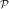and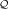on ${B}_{\stackrel{̄}{r}}$ as

$\left(\mathcal{P}x\right)\left(t\right)=\underset{0}{\overset{t}{\int }}\frac{{\left(t-s\right)}^{q-1}}{\mathrm{\Gamma }\left(q\right)}f\left(s,x\left(s\right)\right)ds,$
$\begin{array}{l}\phantom{\rule{1em}{0ex}}\left(\mathcal{Q}x\right)\left(t\right)\phantom{\rule{2em}{0ex}}\\ =\frac{\sigma }{\mathrm{\Delta }\mathrm{\Gamma }\left(q\right)}\left[-\left(\frac{\eta }{2}\left({\delta }^{2}-{\gamma }^{2}\right)-1\right)+t\left(\eta \left(\delta -\gamma \right)-1\right)\right]\underset{\alpha }{\overset{\beta }{\int }}\left(\underset{0}{\overset{s}{\int }}{\left(s-m\right)}^{q-1}f\left(m,x\left(m\right)\right)dm\right)\phantom{\rule{0.3em}{0ex}}\phantom{\rule{0.3em}{0ex}}ds\phantom{\rule{2em}{0ex}}\\ \phantom{\rule{1em}{0ex}}+\frac{\eta }{\mathrm{\Delta }\mathrm{\Gamma }\left(q\right)}\left[\frac{\sigma }{2}\left({\beta }^{2}-{\alpha }^{2}\right)-\left(\sigma \left(\beta -\alpha \right)-1\right)t\right]\underset{\gamma }{\overset{\delta }{\int }}\left(\underset{0}{\overset{s}{\int }}{\left(s-m\right)}^{q-1}f\left(m,x\left(m\right)\right)dm\right)\phantom{\rule{0.3em}{0ex}}\phantom{\rule{0.3em}{0ex}}ds\phantom{\rule{2em}{0ex}}\\ \phantom{\rule{1em}{0ex}}-\frac{1}{\mathrm{\Delta }\mathrm{\Gamma }\left(q\right)}\left[\frac{\sigma }{2}\left({\beta }^{2}-{\alpha }^{2}\right)-\left(\sigma \left(\beta -\alpha \right)-1\right)t\right]\underset{0}{\overset{1}{\int }}{\left(1-s\right)}^{q-1}f\left(s,x\left(s\right)\right)ds.\phantom{\rule{2em}{0ex}}\end{array}$

For $x,y\in {B}_{\stackrel{̄}{r}}$, we find that

$\begin{array}{l}||\mathcal{P}x+\mathcal{Q}y||\\ \le \frac{||\mu ||}{\mathrm{\Gamma }\left(q+1\right)}\left(1+\frac{{\mathrm{\Delta }}_{2}|\sigma |\left({\beta }^{q+1}-{\alpha }^{q+1}\right)+{\mathrm{\Delta }}_{1}|\eta |\left({\delta }^{q+1}-{\gamma }^{q+1}\right)+\left(q+1\right)\phantom{\rule{0.3em}{0ex}}{\mathrm{\Delta }}_{1}}{\left(q+1\right)|\mathrm{\Delta }|}\right)\le \stackrel{̄}{r}.\end{array}$

Thus, $\mathcal{P}x+\mathcal{Q}y\in {B}_{\stackrel{̄}{r}}.$ It follows from the assumption (A1) together with (3.3) thatis a contraction mapping. Continuity of f implies that the operatoris continuous. Also,is uniformly bounded on ${B}_{\stackrel{̄}{r}}$ as

$||\mathcal{P}x||\phantom{\rule{0.3em}{0ex}}\phantom{\rule{0.3em}{0ex}}\le \frac{||\mu ||}{\mathrm{\Gamma }\left(q+1\right)}.$

Now we prove the compactness of the operator.

In view of (A1), we define ${\mathsf{\text{sup}}}_{\left(t,x\right)\in \left[0,1\right]×{B}_{\stackrel{̄}{r}}}|f\left(t,x\right)=\stackrel{̄}{f}$, and consequently we have

which is independent of x. Thus,is equicontinuous. Hence, by the Arzelá-Ascoli Theorem,is compact on ${B}_{\stackrel{̄}{r}}.$ Thus all the assumptions of Theorem 3.2 are satisfied. So the conclusion of Theorem 3.2 implies that the boundary value problem (1.1) has at least one solution on [0, 1]. □

Our next existence result is based on Leray-Schauder degree theory.

Theorem 3.4 Let $f\phantom{\rule{2.77695pt}{0ex}}:\phantom{\rule{2.77695pt}{0ex}}\left[0,1\right]×\phantom{\rule{0.3em}{0ex}}ℝ\to ℝ$. Assume that there exist constants $0\le \kappa <\phantom{\rule{0.3em}{0ex}}\frac{1}{\mathrm{\Lambda }}$, where Λ is given by (3.2) and M > 0 such that |f(t, x)|κ|x|+M for all t [0, 1], x C[0, 1]. Then the boundary value problem (1.1) has at least one solution.

Proof. Consider the fixed point problem

$x=\mathbf{F}x,$
(3.4)

where F is defined by (3.1). In view of the fixed point problem (3.4), we just need to prove the existence of at least one solution x C[0, 1] satisfying (3.4). Define a suitable ball B R C[0, 1] with radius R > 0 as

${B}_{R}=\left\{x\in C\left[0,1\right]:\underset{t\in \left[0,1\right]}{\mathsf{\text{max}}}|x\left(t\right)|

where R will be fixed later. Then, it is sufficient to show that $\mathbf{F}\phantom{\rule{2.77695pt}{0ex}}:{\stackrel{̄}{B}}_{R}\to C\left[0,1\right]$ satisfies

$x\ne \lambda \mathbf{F}x,\phantom{\rule{1em}{0ex}}\forall x\in \partial {B}_{R}\phantom{\rule{1em}{0ex}}and\phantom{\rule{1em}{0ex}}\forall \lambda \in \left[0,1\right].$
(3.5)

Let us set

$H\left(\lambda ,x\right)=\lambda \mathbf{F}x,\phantom{\rule{1em}{0ex}}x\in C\left(ℝ\right)\phantom{\rule{1em}{0ex}}\lambda \in \left[0,1\right].$

Then, by the Arzelá-Ascoli Theorem, h λ (x) = x - H (λ, x) = x - λF x is completely continuous. If (3.5) is true, then the following Leray-Schauder degrees are well defined and by the homotopy invariance of topological degree, it follows that

$\begin{array}{ll}\hfill \mathsf{\text{deg}}\left({h}_{\lambda },\phantom{\rule{2.77695pt}{0ex}}{B}_{R},\phantom{\rule{2.77695pt}{0ex}}0\right)& =\mathsf{\text{deg}}\left(I-\lambda F,\phantom{\rule{2.77695pt}{0ex}}{B}_{R},\phantom{\rule{2.77695pt}{0ex}}0\right)=\mathsf{\text{deg}}\left({h}_{1},\phantom{\rule{2.77695pt}{0ex}}{B}_{R},\phantom{\rule{2.77695pt}{0ex}}0\right)\phantom{\rule{2em}{0ex}}\\ =\mathsf{\text{deg}}\left({h}_{0},\phantom{\rule{2.77695pt}{0ex}}{B}_{R},\phantom{\rule{2.77695pt}{0ex}}0\right)=\mathsf{\text{deg}}\left(I,\phantom{\rule{2.77695pt}{0ex}}{B}_{R},\phantom{\rule{2.77695pt}{0ex}}0\right)=1\ne 0,\phantom{\rule{2.77695pt}{0ex}}0\in {B}_{r},\phantom{\rule{2em}{0ex}}\end{array}$

where I denotes the unit operator. By the nonzero property of Leray-Schauder degree, h1(t) = x - λ F x = 0 for at least one x B R . In order to prove (3.5), we assume that x = λ F x, λ [0, 1]. Then for x ∂B R and t [0, 1] we have

which, on taking norm $\left({\mathsf{\text{sup}}}_{t\in \left[0,1\right]}\left|x\left(t\right)\right|=∥x∥\right)$ and solving for x, yields

$||x||\phantom{\rule{0.3em}{0ex}}\phantom{\rule{0.3em}{0ex}}\le \frac{M\mathrm{\Lambda }}{1-\kappa \mathrm{\Lambda }}.$

Letting $R=\frac{M\mathrm{\Lambda }}{1-\kappa \mathrm{\Lambda }}+1$, (3.5) holds. This completes the proof. □

Example 3.5 Consider the following strip fractional boundary value problem

$\left\{\begin{array}{c}\hfill {}^{c}{D}^{3/2}x\left(t\right)=\frac{1}{{\left(t+2\right)}^{2}}\frac{|x|}{1+|x|},t\in \left[0,1\right],\hfill \\ \hfill x\left(0\right)=\underset{1/3}{\overset{1/2}{\int }}x\left(s\right)ds,x\left(1\right)=\underset{2/3}{\overset{3/4}{\int }}x\left(s\right)ds.\hfill \end{array}\right\$
(3.6)

Here, q = 3/2, σ = 1, η = 1, α = 1/3, β = 1/2, γ = 2/3, δ = 3/4 and $f\left(t,x\right)=\frac{1}{{\left(t+2\right)}^{2}}\frac{|x|}{1+|x|}$. As $|f\left(t,x\right)-f\left(t,y\right)|\phantom{\rule{0.3em}{0ex}}\phantom{\rule{0.3em}{0ex}}\le \frac{1}{4}|x-y|$, therefore, (A1) is satisfied with $L=\frac{1}{4}$. Further, Δ1 = 65/72, Δ2 = 535/288, Δ = 4945/5184, and

$\mathrm{\Lambda }=\frac{1}{\mathrm{\Gamma }\left(q+1\right)}\left(1+\frac{{\mathrm{\Delta }}_{2}|\sigma |\left({\beta }^{q+1}-{\alpha }^{q+1}\right)+{\mathrm{\Delta }}_{1}|\eta |\left({\delta }^{q+1}-{\gamma }^{q+1}\right)+\left(q+1\right){\mathrm{\Delta }}_{1}}{\left(q+1\right)|\mathrm{\Delta }|}\right)=1.128765.$

Clearly, L Λ = 0.282191 < 1. Thus, by the conclusion of Theorem 3.1, the boundary value problem (3.6) has a unique solution on [0, 1].

Example 3.6 Consider the following boundary value problem

$\left\{\begin{array}{l}{}^{c}{D}^{3/2}x\left(t\right)=\frac{1}{\left(4\pi \right)}\mathsf{\text{sin}}\left(2\pi x\right)+\frac{|x|}{1+|x|},\phantom{\rule{2.77695pt}{0ex}}\phantom{\rule{1em}{0ex}}t\in \left[0,1\right],\phantom{\rule{2.77695pt}{0ex}}\phantom{\rule{1em}{0ex}}1
(3.7)

Here,

$|f\left(t,x\right)|=\left|\frac{1}{\left(4\pi \right)}\mathsf{\text{sin}}\left(2\pi x\right)+\frac{|x|}{1+|x|}\right|\le \frac{1}{2}|x|+1.$

Clearly M = 1 and

$\kappa =\frac{1}{2}<\frac{1}{\mathrm{\Lambda }}=0.885924.$

Thus, all the conditions of Theorem 3.4 are satisfied and consequently the problem (3.7) has at least one solution.

## 4 Existence results for multi-valued case

### 4.1 Preliminaries

Let us recall some basic definitions on multi-valued maps [28, 29].

For a normed space (X, .), let ${P}_{cl}\left(X\right)=\left\{Y\in \mathcal{P}\left(X\right)\phantom{\rule{2.77695pt}{0ex}}:\phantom{\rule{2.77695pt}{0ex}}Y\phantom{\rule{2.77695pt}{0ex}}\mathsf{\text{is}}\phantom{\rule{0.3em}{0ex}}\mathsf{\text{closed}}\right\}$, ${P}_{b}\left(X\right)=\left\{Y\in \mathcal{P}\left(X\right)\phantom{\rule{2.77695pt}{0ex}}:\phantom{\rule{2.77695pt}{0ex}}Y\phantom{\rule{2.77695pt}{0ex}}\mathsf{\text{is}}\phantom{\rule{0.3em}{0ex}}\mathsf{\text{bounded}}\right\}$, ${P}_{cp}\left(X\right)=\left\{Y\in \mathcal{P}\left(X\right)\phantom{\rule{2.77695pt}{0ex}}:\phantom{\rule{2.77695pt}{0ex}}Y\phantom{\rule{2.77695pt}{0ex}}\mathsf{\text{is}}\phantom{\rule{0.3em}{0ex}}\mathsf{\text{compact}}\right\}$, and ${P}_{cp,c}\left(X\right)=\left\{Y\in \mathcal{P}\left(X\right)\phantom{\rule{2.77695pt}{0ex}}:\phantom{\rule{2.77695pt}{0ex}}Y\phantom{\rule{2.77695pt}{0ex}}\mathsf{\text{is}}\phantom{\rule{0.3em}{0ex}}\mathsf{\text{compact}}\phantom{\rule{0.3em}{0ex}}\mathsf{\text{and}}\phantom{\rule{0.3em}{0ex}}\mathsf{\text{convex}}\right\}$. A multi-valued map $G\phantom{\rule{2.77695pt}{0ex}}:\phantom{\rule{2.77695pt}{0ex}}X\to \mathcal{P}\left(X\right)$is convex (closed) valued if G(x) is convex (closed) for all x X. The map G is bounded on bounded sets if $G\left(\mathbb{B}\right)={\cup }_{x\in \mathbb{B}}G\left(x\right)$ is bounded in X for all $\mathbb{B}\in {P}_{b}\left(X\right)$ (i.e., ${\mathsf{\text{sup}}}_{x\in \mathbb{B}}\left\{\mathsf{\text{sup}}\left\{|y|:y\in G\left(x\right)\right\}\right\}<\infty \right)$. G is called upper semi-continuous (u.s.c.) on X if for each x0 X, the set G(x0) is a nonempty closed subset of X, and if for each open set N of X containing G(x0), there exists an open neighborhood ${\mathcal{N}}_{0}$ of x0 such that $G\left({\mathcal{N}}_{0}\right)\subseteq N$. G is said to be completely continuous if $G\left(\mathbb{B}\right)$ is relatively compact for every $\mathbb{B}\in {P}_{b}\left(X\right)$. If the multi-valued map G is completely continuous with nonempty compact values, then G is u.s.c. if and only if G has a closed graph, i.e., x n x*, y n y*, y n G(x n ) imply y* G(x*). G has a fixed point if there is x X such that x G(x). The fixed point set of the multivalued operator G will be denoted by FixG. A multivalued map $G\phantom{\rule{2.77695pt}{0ex}}:\phantom{\rule{2.77695pt}{0ex}}\left[0;1\right]\to {P}_{cl}\left(ℝ\right)$ is said to be measurable if for every $y\in ℝ$, the function

$t↦d\left(y,G\left(t\right)\right)=\mathsf{\text{inf}}\left\{|y-z|\phantom{\rule{2.77695pt}{0ex}}:\phantom{\rule{2.77695pt}{0ex}}z\in G\left(t\right)\right\}$

is measurable.

Let C([0, 1]) denotes a Banach space of continuous functions from [0, 1] into with the norm $∥x∥={\mathsf{\text{sup}}}_{t\in \left[0,1\right]}\left|x\left(t\right)\right|$. Let L1([0, 1], ) be the Banach space of measurable functions x : [0, 1] → which are Lebesgue integrable and normed by ${∥x∥}_{{L}^{1}}={\int }_{0}^{1}|x\left(t\right)|dt$.

Definition 4.1 A multivalued map $F\phantom{\rule{2.77695pt}{0ex}}:\phantom{\rule{2.77695pt}{0ex}}\left[0,1\right]×ℝ\to \mathcal{P}\left(ℝ\right)$ is said to be Carathéodory if

(i) t F (t, x) is measurable for each x ;

(ii) x F (t, x) is upper semicontinuous for almost all t [0, 1];

Further a Carathéodory function F is called L 1 -Carathéodory if

(iii) for each α > 0, there exists ${\phi }_{\alpha }\in {L}^{1}\left(\left[0,1\right],{ℝ}^{+}\right)$ such that

$||F\left(t,x\right)||\phantom{\rule{0.3em}{0ex}}=\mathsf{\text{sup}}\left\{|v|\phantom{\rule{2.77695pt}{0ex}}:\phantom{\rule{2.77695pt}{0ex}}v\in F\left(t,x\right)\right\}\le {\phi }_{\alpha }\left(t\right)$

for all xα and for a. e. t [0, 1].

For each $y\in C\left(\left[0,1\right],ℝ\right)$, define the set of selections of F by

${S}_{F,y}:=\left\{v\in {L}^{1}\left(\left[0,1\right],ℝ\right):v\left(t\right)\in F\left(t,y\left(t\right)\right)\phantom{\rule{0.3em}{0ex}}\mathsf{\text{for}}\phantom{\rule{0.3em}{0ex}}\mathsf{\text{a}}\mathsf{\text{.e}}\mathsf{\text{.}}\phantom{\rule{0.3em}{0ex}}t\in \left[0,1\right]\right\}.$

Let X be a nonempty closed subset of a Banach space E and $G\phantom{\rule{2.77695pt}{0ex}}:\phantom{\rule{2.77695pt}{0ex}}X\to \mathcal{P}\left(E\right)$ be a multivalued operator with nonempty closed values. G is lower semi-continuous (l.s.c.) if the set {y X : G(y) ∩ B} is open for any open set B in E. Let A be a subset of [0, 1] × . A is $\mathcal{L}\otimes \mathcal{B}$ measurable if A belongs to the σ-algebra generated by all sets of the form $\mathcal{J}×\mathcal{D}$, where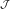is Lebesgue measurable in [0, 1] and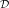is Borel measurable in . A subset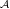of L1([0, 1], ) is decomposable if for all $u,v\in \mathcal{A}$ and measurable $\mathcal{J}\subset \left[0,1\right]=J$, the function $u{\chi }_{\mathcal{J}}+v{\chi }_{J-\mathcal{J}}\in \mathcal{A}$, where ${\chi }_{\mathcal{J}}$ stands for the characteristic function of.

Definition 4.2 Let Y be a separable metric space and let $N\phantom{\rule{2.77695pt}{0ex}}:\phantom{\rule{2.77695pt}{0ex}}Y\to \mathcal{P}\left({L}^{1}\left(\left[0,1\right],ℝ\right)\right)$ be a multivalued operator. We say N has a property (BC) if N is lower semi-continuous (l.s.c.) and has nonempty closed and decomposable values.

Let $F\phantom{\rule{2.77695pt}{0ex}}:\phantom{\rule{2.77695pt}{0ex}}\left[0,1\right]×ℝ\to \mathcal{P}\left(ℝ\right)$ be a multivalued map with nonempty compact values. Define a multivalued operator $\mathcal{F}\phantom{\rule{2.77695pt}{0ex}}:\phantom{\rule{2.77695pt}{0ex}}C\left(\left[0,1\right]×ℝ\right)\to \mathcal{P}\left({L}^{1}\left(\left[0,1\right],ℝ\right)\right)$ associated with F as

$\mathcal{F}\left(x\right)=\left\{w\in {L}^{1}\left(\left[0,1\right],ℝ\right):w\left(t\right)\in F\left(t,x\left(t\right)\right)\phantom{\rule{0.3em}{0ex}}\phantom{\rule{0.3em}{0ex}}\mathsf{\text{for}}\phantom{\rule{0.3em}{0ex}}\mathsf{\text{a}}\mathsf{\text{.e}}\mathsf{\text{.}}\phantom{\rule{0.3em}{0ex}}t\in \left[0,1\right]\right\},$

which is called the Nemytskii operator associated with F.

Definition 4.3 Let $F\phantom{\rule{2.77695pt}{0ex}}:\phantom{\rule{2.77695pt}{0ex}}\left[0,1\right]×ℝ\to \mathcal{P}\left(ℝ\right)$ be a multivalued function with nonempty compact values. We say F is of lower semi-continuous type (l.s.c. type) if its associated Nemytskii operator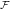is lower semi-continuous and has nonempty closed and decomposable values.

Let (X, d) be a metric space induced from the normed space (X; .). Consider ${H}_{d}:\mathcal{P}\left(X\right)×\mathcal{P}\left(X\right)\to ℝ\cup \left\{\infty \right\}$ given by

${H}_{d}\left(A,B\right)=\mathsf{\text{max}}\left\{\underset{a\in A}{\mathsf{\text{sup}}}d\left(a,B\right),\phantom{\rule{2.77695pt}{0ex}}\underset{b\in B}{\mathsf{\text{sup}}}d\left(A,b\right)\right\},$

where d(A, b) = infaAd(a; b) and d(a, B) = infbBd(a; b). Then (P b,cl (X), H d ) is a metric space and (P cl (X), H d ) is a generalized metric space (see ).

Definition 4.4 A multivalued operator N : XP cl (X) is called:

(a) γ-Lipschitz if and only if there exists γ > 0 such that

${H}_{d}\left(N\left(x\right),N\left(y\right)\right)\le \gamma d\left(x,y\right)\phantom{\rule{2.77695pt}{0ex}}for\phantom{\rule{2.77695pt}{0ex}}each\phantom{\rule{2.77695pt}{0ex}}x,y\in X;$

(b) a contraction if and only if it is γ-Lipschitz with γ < 1.

The following lemmas will be used in the sequel.

Lemma 4.5 (Nonlinear alternative for Kakutani maps) . Let E be a Banach space, C is a closed convex subset of E, U is an open subset of C and 0 U. Suppose that $F:\overline{U}\to {\mathcal{P}}_{c,cv}\left(C\right)$ is a upper semicontinuous compact map; here ${\mathcal{P}}_{c,cv}\left(C\right)$ denotes the family of nonempty, compact convex subsets of C. Then either

(i) F has a fixed point in $\overline{U}$, or

(ii) there is a u ∂U and λ (0, 1) with u λF(u).

Lemma 4.6  Let X be a Banach space. Let $F\phantom{\rule{2.77695pt}{0ex}}:\phantom{\rule{2.77695pt}{0ex}}\left[0,T\right]×ℝ\to {\mathcal{P}}_{cp,c}\left(X\right)$ be an L1-Carathéodory multivalued map and let θ be a linear continuous mapping from L1([0, 1], X) to C([0, 1], X). Then the operator

$\mathrm{\Theta }\circ {S}_{F}:C\left(\left[0,1\right],X\right)\to {P}_{cp,c}\left(C\left(\left[0,1\right],X\right)\right),\phantom{\rule{2.77695pt}{0ex}}\phantom{\rule{1em}{0ex}}x↦\left(\mathrm{\Theta }\circ {S}_{F}\right)\left(x\right)=\mathrm{\Theta }\left({S}_{F,x}\right)$

is a closed graph operator in C([0, 1], X) × C([0, 1], X).

Lemma 4.7  Let Y be a separable metric space and let $N:Y\to \mathcal{P}\left({L}^{1}\left(\left[0,1\right],ℝ\right)\right)$ be a multivalued operator satisfying the property (BC). Then N has a continuous selection, that is, there exists a continuous function (single-valued) $g:Y\to {L}^{1}\left(\left[0,1\right],ℝ\right)$ such that g(x) N(x) for every x Y .

Lemma 4.8  Let (X, d) be a complete metric space. If N : XP cl (X) is a contraction, then FixN ≠ .

Definition 4.9 A function x C2([0, 1], ) is a solution of the problem (1.2) if $x\left(0\right)=\sigma \underset{\alpha }{\overset{\beta }{\int }}x\left(s\right)ds,\phantom{\rule{0.3em}{0ex}}x\left(1\right)=\eta \underset{\gamma }{\overset{\delta }{\int }}x\left(s\right)ds$, and there exists a function f L1([0, 1], ) such that

f(t) F (t, x(t)) a.e. on [0, 1] and

$\begin{array}{ll}\hfill x\left(t\right)& =\frac{1}{\mathrm{\Gamma }\left(q\right)}\underset{0}{\overset{t}{\int }}{\left(t-s\right)}^{q-1}f\left(s\right)ds\phantom{\rule{2em}{0ex}}\\ +\frac{\sigma }{\mathrm{\Delta }\mathrm{\Gamma }\left(q\right)}\left[-\left(\frac{\eta }{2}\left({\delta }^{2}-{\gamma }^{2}\right)-1\right)+t\left(\eta \left(\delta -\gamma \right)-1\right)\right]\underset{\alpha }{\overset{\beta }{\int }}\left(\underset{0}{\overset{s}{\int }}{\left(s-m\right)}^{q-1}f\left(m\right)dm\right)\phantom{\rule{0.3em}{0ex}}\phantom{\rule{0.3em}{0ex}}ds\phantom{\rule{2em}{0ex}}\\ +\frac{\eta }{\mathrm{\Delta }\mathrm{\Gamma }\left(q\right)}\left[\frac{\sigma }{2}\left({\beta }^{2}-{\alpha }^{2}\right)-\left(\sigma \left(\beta -\alpha \right)-1\right)t\right]\underset{\gamma }{\overset{\delta }{\int }}\left(\underset{0}{\overset{s}{\int }}{\left(s-m\right)}^{q-1}f\left(m\right)dm\right)\phantom{\rule{0.3em}{0ex}}\phantom{\rule{0.3em}{0ex}}ds\phantom{\rule{2em}{0ex}}\\ -\frac{1}{\mathrm{\Delta }\mathrm{\Gamma }\left(q\right)}\left[\frac{\sigma }{2}\left({\beta }^{2}-{\alpha }^{2}\right)-\left(\sigma \left(\beta -\alpha \right)-1\right)t\right]\underset{0}{\overset{1}{\int }}{\left(1-s\right)}^{q-1}f\left(s\right)ds.\phantom{\rule{2em}{0ex}}\end{array}$
(4.1)

### 4.2 The Carathéodory case

Theorem 4.10 Assume that:

(H1) $F\phantom{\rule{2.77695pt}{0ex}}:\phantom{\rule{2.77695pt}{0ex}}\left[0,1\right]×ℝ\to \mathcal{P}\left(ℝ\right)$ is Carathéodory and has nonempty compact and convex values;

(H2) there exists a continuous nondecreasing function ψ : [0, ∞) → (0, ∞) and a function $p\in {L}^{1}\left(\left[0,1\right],{ℝ}^{+}\right)$ such that

$||F\left(t,x\right)|{|}_{\mathcal{P}}:=\mathsf{\text{sup}}\left\{|y|\phantom{\rule{2.77695pt}{0ex}}:\phantom{\rule{2.77695pt}{0ex}}y\in F\left(t,x\right)\right\}\le p\left(t\right)\psi \left(||x||\right)\phantom{\rule{1em}{0ex}}\phantom{\rule{0.3em}{0ex}}for\phantom{\rule{0.3em}{0ex}}each\phantom{\rule{1em}{0ex}}\left(t,x\right)\in \left[0,1\right]\phantom{\rule{0.3em}{0ex}}×\phantom{\rule{0.3em}{0ex}}ℝ.\phantom{\rule{2.77695pt}{0ex}}$

(H3) there exists a constant M > 0 such that

Then the boundary value problem (1.2) has at least one solution on [0, 1].

Proof. Define the operator ${\mathrm{\Omega }}_{F}:C\left(\left[0,1\right],ℝ\right)\to \mathcal{P}\left(C\left(\left[0,1\right],ℝ\right)\right)$ by

${\mathrm{\Omega }}_{F}\left(x\right)=\left\{\begin{array}{l}h\in C\left(\left[0,1\right],ℝ\right):\\ h\left(t\right)=\left\{\begin{array}{l}\frac{1}{\mathrm{\Gamma }\left(q\right)}\underset{0}{\overset{t}{\int }}{\left(t-s\right)}^{q-1}f\left(s\right)ds\\ +\frac{\sigma }{\mathrm{\Delta }\mathrm{\Gamma }\left(q\right)}\left[-\left(\frac{\eta }{2}\left({\delta }^{2}-{\gamma }^{2}\right)-1\right)+t\left(\eta \left(\delta -\gamma \right)-1\right)\right]×\\ ×\underset{\alpha }{\overset{\beta }{\int }}\left(\underset{0}{\overset{s}{\int }}{\left(s-m\right)}^{q-1}f\left(m\right)dm\right)\phantom{\rule{0.3em}{0ex}}\phantom{\rule{0.3em}{0ex}}ds\\ +\frac{\eta }{\mathrm{\Delta }\mathrm{\Gamma }\left(q\right)}\left[\frac{\sigma }{2}\left({\beta }^{2}-{\alpha }^{2}\right)-\left(\sigma \left(\beta -\alpha \right)-1\right)t\right]×\\ ×\underset{\gamma }{\overset{\delta }{\int }}\left(\underset{0}{\overset{s}{\int }}{\left(s-m\right)}^{q-1}f\left(m\right)dm\right)\phantom{\rule{0.3em}{0ex}}\phantom{\rule{0.3em}{0ex}}ds\\ -\frac{1}{\mathrm{\Delta }\mathrm{\Gamma }\left(q\right)}\left[\frac{\sigma }{2}\left({\beta }^{2}-\phantom{\rule{0.3em}{0ex}}{\alpha }^{2}\right)-\left(\sigma \left(\beta -\alpha \right)-1\right)t\right]\underset{0}{\overset{1}{\int }}{\left(1-s\right)}^{q-1}f\left(s\right)ds,\end{array}\right\\end{array}\right\}$

for f S F,x . We will show that Ω F satisfies the assumptions of the nonlinear alternative of Leray-Schauder type. The proof consists of several steps. As a first step, we show that Ω F is convex for each x C([0, 1], ). This step is obvious since S F,x is convex (F has convex values), and therefore we omit the proof.

In the second step, we show that Ω F maps bounded sets (balls) into bounded sets in C([0, 1], ). For a positive number ρ, let B ρ = {x C([0, 1], ): xρ} be a bounded ball in C([0, 1], ). Then, for each h Ω F (x), x B ρ , there exists f S F,x such that

$\begin{array}{l}h\left(t\right)=\frac{1}{\mathrm{\Gamma }\left(q\right)}\underset{0}{\overset{t}{\int }}{\left(t-s\right)}^{q-1}f\left(s\right)ds\phantom{\rule{2em}{0ex}}\\ +\frac{\sigma }{\mathrm{\Delta }\mathrm{\Gamma }\left(q\right)}\left[-\left(\frac{\eta }{2}\left({\delta }^{2}-{\gamma }^{2}\right)-1\right)+t\left(\eta \left(\delta -\gamma \right)-1\right)\right]\underset{\alpha }{\overset{\beta }{\int }}\left(\underset{0}{\overset{s}{\int }}{\left(s-m\right)}^{q-1}f\left(m\right)dm\right)\phantom{\rule{0.3em}{0ex}}\phantom{\rule{0.3em}{0ex}}ds\phantom{\rule{2em}{0ex}}\\ +\frac{\eta }{\mathrm{\Delta }\mathrm{\Gamma }\left(q\right)}\left[\frac{\sigma }{2}\left({\beta }^{2}-{\alpha }^{2}\right)-\left(\sigma \left(\beta -\alpha \right)-1\right)t\right]\underset{\gamma }{\overset{\delta }{\int }}\left(\underset{0}{\overset{s}{\int }}{\left(s-m\right)}^{q-1}f\left(m\right)dm\right)\phantom{\rule{0.3em}{0ex}}ds\phantom{\rule{2em}{0ex}}\\ -\frac{1}{\mathrm{\Delta }\mathrm{\Gamma }\left(q\right)}\left[\frac{\sigma }{2}\left({\beta }^{2}-{\alpha }^{2}\right)-\left(\sigma \left(\beta -\alpha \right)-1\right)t\right]\underset{0}{\overset{1}{\int }}{\left(1-s\right)}^{q-1}f\left(s\right)ds.\phantom{\rule{2em}{0ex}}\end{array}$

Then for t[0, 1] we have

Thus,

Now we show that Ω F maps bounded sets into ;equicontinuous sets of C([0, 1], ).

Let t', t'' [0, 1] with t' < t'' and x B ρ . For each h Ω F (x), we obtain

$\begin{array}{l}\phantom{\rule{1em}{0ex}}\left|h\left({t}^{″}\right)\phantom{\rule{0.3em}{0ex}}-\phantom{\rule{0.3em}{0ex}}h\left({t}^{\prime }\right)\right|\phantom{\rule{2em}{0ex}}\\ \le \phantom{\rule{0.3em}{0ex}}\left|\psi \left(∥x∥\right)\phantom{\rule{0.3em}{0ex}}\underset{0}{\overset{{t}^{\prime }}{\int }}\left[\frac{{\left({t}^{″}\phantom{\rule{0.3em}{0ex}}-\phantom{\rule{0.3em}{0ex}}s\right)}^{q-1}\phantom{\rule{0.3em}{0ex}}-\phantom{\rule{0.3em}{0ex}}{\left({t}^{\prime }\phantom{\rule{0.3em}{0ex}}-\phantom{\rule{0.3em}{0ex}}s\right)}^{q-1}}{\mathrm{\Gamma }\left(q\right)}\right]\phantom{\rule{0.3em}{0ex}}p\left(s\right)ds\phantom{\rule{0.3em}{0ex}}+\phantom{\rule{0.3em}{0ex}}\psi \left(∥x∥\right)\underset{{t}^{\prime }}{\overset{{t}^{″}}{\int }}\phantom{\rule{0.3em}{0ex}}\frac{{\left({t}^{″}\phantom{\rule{0.3em}{0ex}}-\phantom{\rule{0.3em}{0ex}}s\right)}^{q-1}}{\mathrm{\Gamma }\left(q\right)}p\left(s\right)ds\right|\phantom{\rule{2em}{0ex}}\\ \phantom{\rule{1em}{0ex}}+\left|\frac{\psi \left(∥x∥\right)\sigma }{\mathrm{\Delta }\mathrm{\Gamma }\left(q\right)}\left(\eta \left(\delta \phantom{\rule{0.3em}{0ex}}-\phantom{\rule{0.3em}{0ex}}\gamma \right)\phantom{\rule{0.3em}{0ex}}-\phantom{\rule{0.3em}{0ex}}1\right)\left|{t}^{″}\phantom{\rule{0.3em}{0ex}}-\phantom{\rule{0.3em}{0ex}}{t}^{\prime }\right|\phantom{\rule{0.3em}{0ex}}\right|\phantom{\rule{0.3em}{0ex}}\underset{\alpha }{\overset{\beta }{\int }}\left(\underset{0}{\overset{s}{\int }}{\left(s\phantom{\rule{0.3em}{0ex}}-\phantom{\rule{0.3em}{0ex}}m\right)}^{q-1}p\left(m\right)\phantom{\rule{0.3em}{0ex}}dm\right)\phantom{\rule{0.3em}{0ex}}ds\phantom{\rule{2em}{0ex}}\\ \phantom{\rule{1em}{0ex}}+\left|\frac{\eta \psi \left(∥x∥\right)}{\mathrm{\Delta }\mathrm{\Gamma }\left(q\right)}\left[\left(\sigma \left(\beta \phantom{\rule{0.3em}{0ex}}-\phantom{\rule{0.3em}{0ex}}\alpha \right)+1\right)\left|{t}^{″}\phantom{\rule{0.3em}{0ex}}-\phantom{\rule{0.3em}{0ex}}{t}^{\prime }\right|\right]\right|\phantom{\rule{0.3em}{0ex}}\underset{\gamma }{\overset{\delta }{\int }}\left(\underset{0}{\overset{s}{\int }}{\left(s\phantom{\rule{0.3em}{0ex}}-\phantom{\rule{0.3em}{0ex}}m\right)}^{q-1}p\left(m\right)dm\right)\phantom{\rule{0.3em}{0ex}}ds\phantom{\rule{2em}{0ex}}\\ \phantom{\rule{1em}{0ex}}+\phantom{\rule{0.3em}{0ex}}\left|\frac{\psi \left(∥x∥\right)}{\mathrm{\Delta }\mathrm{\Gamma }\left(q\right)}\left[\left(\sigma \left(\beta \phantom{\rule{0.3em}{0ex}}-\phantom{\rule{0.3em}{0ex}}\alpha \right)\phantom{\rule{0.3em}{0ex}}+\phantom{\rule{0.3em}{0ex}}1\right)\phantom{\rule{0.3em}{0ex}}\left|{t}^{″}\phantom{\rule{0.3em}{0ex}}-\phantom{\rule{0.3em}{0ex}}{t}^{\prime }\right|\right]\phantom{\rule{0.3em}{0ex}}\right|\phantom{\rule{0.3em}{0ex}}\underset{0}{\overset{1}{\int }}{\left(1\phantom{\rule{0.3em}{0ex}}-\phantom{\rule{0.3em}{0ex}}s\right)}^{q-1}\phantom{\rule{0.3em}{0ex}}p\left(s\right)\phantom{\rule{0.3em}{0ex}}ds.\phantom{\rule{2em}{0ex}}\end{array}$

Obviously the right-hand side of the above inequality tends to zero independently of x B ρ as t'' - t' → 0. As Ω F satisfies the above three assumptions, therefore it follows by the Ascoli-Arzelá theorem that ${\mathrm{\Omega }}_{F}:C\left(\left[0,1\right],ℝ\right)\to \mathcal{P}\left(C\left(\left[0,1\right],ℝ\right)\right)$ is completely continuous.

In our next step, we show that Ω F has a closed graph. Let ${x}_{n}\phantom{\rule{0.3em}{0ex}}\to \phantom{\rule{0.3em}{0ex}}{x}_{*},\phantom{\rule{0.3em}{0ex}}{h}_{n}\phantom{\rule{0.3em}{0ex}}\in \phantom{\rule{0.3em}{0ex}}{\mathrm{\Omega }}_{F}\left({x}_{n}\right)$, and ${h}_{n}\phantom{\rule{0.3em}{0ex}}\to \phantom{\rule{0.3em}{0ex}}{h}_{*}$. Then we need to show that ${h}_{*\phantom{\rule{0.3em}{0ex}}}\in \phantom{\rule{0.3em}{0ex}}\phantom{\rule{0.3em}{0ex}}{\mathrm{\Omega }}_{F}\left({x}_{*}\right)$. Associated with h n Ω F (x n ), there exists ${f}_{n}\phantom{\rule{0.3em}{0ex}}\in \phantom{\rule{0.3em}{0ex}}{S}_{F,{x}_{n}}$ such that for each t [0, 1],

$\begin{array}{ll}\hfill {h}_{n}\left(t\right)\phantom{\rule{0.3em}{0ex}}& =\phantom{\rule{0.3em}{0ex}}\frac{1}{\mathrm{\Gamma }\left(q\right)}\phantom{\rule{0.3em}{0ex}}\underset{0}{\overset{t}{\int }}{\left(t\phantom{\rule{0.3em}{0ex}}-\phantom{\rule{0.3em}{0ex}}s\right)}^{q-1}{f}_{n}\left(s\right)\phantom{\rule{0.3em}{0ex}}ds\phantom{\rule{2em}{0ex}}\\ \phantom{\rule{1em}{0ex}}+\phantom{\rule{0.3em}{0ex}}\frac{\sigma }{\mathrm{\Delta }\mathrm{\Gamma }\left(q\right)}\phantom{\rule{0.3em}{0ex}}\left[-\phantom{\rule{0.3em}{0ex}}\left(\frac{\eta }{2}\left({\delta }^{2}\phantom{\rule{0.3em}{0ex}}-\phantom{\rule{0.3em}{0ex}}{\gamma }^{2}\right)\phantom{\rule{0.3em}{0ex}}-\phantom{\rule{0.3em}{0ex}}1\right)\phantom{\rule{0.3em}{0ex}}+\phantom{\rule{0.3em}{0ex}}t\left(\eta \left(\delta \phantom{\rule{0.3em}{0ex}}-\phantom{\rule{0.3em}{0ex}}\gamma \right)\phantom{\rule{0.3em}{0ex}}-\phantom{\rule{0.3em}{0ex}}1\right)\right]\phantom{\rule{0.3em}{0ex}}\underset{\alpha }{\overset{\beta }{\int }}\left(\underset{0}{\overset{s}{\int }}{\left(s\phantom{\rule{0.3em}{0ex}}-\phantom{\rule{0.3em}{0ex}}m\right)}^{q-1}{f}_{n}\left(m\right)\phantom{\rule{0.3em}{0ex}}dm\right)\phantom{\rule{0.3em}{0ex}}ds\phantom{\rule{2em}{0ex}}\\ \phantom{\rule{1em}{0ex}}+\phantom{\rule{0.3em}{0ex}}\frac{\eta }{\mathrm{\Delta }\mathrm{\Gamma }\left(q\right)}\left[\frac{\sigma }{2}\left({\beta }^{2}\phantom{\rule{0.3em}{0ex}}-\phantom{\rule{0.3em}{0ex}}{\alpha }^{2}\right)\phantom{\rule{0.3em}{0ex}}-\phantom{\rule{0.3em}{0ex}}\left(\sigma \left(\beta \phantom{\rule{0.3em}{0ex}}-\phantom{\rule{0.3em}{0ex}}\alpha \right)-\phantom{\rule{0.3em}{0ex}}1\right)t\right]\phantom{\rule{0.3em}{0ex}}\phantom{\rule{0.3em}{0ex}}\underset{\gamma }{\overset{\delta }{\int }}\left(\underset{0}{\overset{s}{\int }}{\left(s\phantom{\rule{0.3em}{0ex}}-\phantom{\rule{0.3em}{0ex}}m\right)}^{q-1}{f}_{n}\left(m\right)dm\right)\phantom{\rule{0.3em}{0ex}}ds\phantom{\rule{2em}{0ex}}\\ \phantom{\rule{1em}{0ex}}-\phantom{\rule{0.3em}{0ex}}\frac{1}{\mathrm{\Delta }\mathrm{\Gamma }\left(q\right)}\phantom{\rule{0.3em}{0ex}}\left[\frac{\sigma }{2}\left({\beta }^{2}\phantom{\rule{0.3em}{0ex}}-\phantom{\rule{0.3em}{0ex}}{\alpha }^{2}\right)\phantom{\rule{0.3em}{0ex}}-\phantom{\rule{0.3em}{0ex}}\left(\sigma \left(\beta \phantom{\rule{0.3em}{0ex}}-\phantom{\rule{0.3em}{0ex}}\alpha \right)\phantom{\rule{0.3em}{0ex}}-\phantom{\rule{0.3em}{0ex}}1\right)\phantom{\rule{0.3em}{0ex}}t\right]\phantom{\rule{0.3em}{0ex}}\underset{0}{\overset{1}{\int }}{\left(1\phantom{\rule{0.3em}{0ex}}-\phantom{\rule{0.3em}{0ex}}s\right)}^{q-1}\phantom{\rule{0.3em}{0ex}}{f}_{n}\left(s\right)\phantom{\rule{0.3em}{0ex}}ds,\phantom{\rule{2em}{0ex}}\end{array}$

Thus it suffices to show that there exists ${f}_{*}\phantom{\rule{0.3em}{0ex}}\in \phantom{\rule{0.3em}{0ex}}{S}_{F,{x}_{*}}$ such that for each t [0, 1],

$\begin{array}{ll}\hfill {h}_{*}\left(t\right)\phantom{\rule{0.3em}{0ex}}& =\phantom{\rule{0.3em}{0ex}}\frac{1}{\mathrm{\Gamma }\left(q\right)}\phantom{\rule{0.3em}{0ex}}\underset{0}{\overset{t}{\int }}{\left(t\phantom{\rule{0.3em}{0ex}}-\phantom{\rule{0.3em}{0ex}}s\right)}^{q-1}{f}_{{}_{*}}\left(s\right)\phantom{\rule{0.3em}{0ex}}ds\phantom{\rule{2em}{0ex}}\\ \phantom{\rule{1em}{0ex}}+\phantom{\rule{0.3em}{0ex}}\frac{\sigma }{\mathrm{\Delta }\mathrm{\Gamma }\left(q\right)}\left[-\left(\frac{\eta }{2}\left({\delta }^{2}\phantom{\rule{0.3em}{0ex}}-\phantom{\rule{0.3em}{0ex}}{\gamma }^{2}\right)-1\right)\phantom{\rule{0.3em}{0ex}}+\phantom{\rule{0.3em}{0ex}}t\left(\eta \left(\delta \phantom{\rule{0.3em}{0ex}}-\phantom{\rule{0.3em}{0ex}}\gamma \right)\phantom{\rule{0.3em}{0ex}}-\phantom{\rule{0.3em}{0ex}}1\right)\right]\phantom{\rule{0.3em}{0ex}}\underset{\alpha }{\overset{\beta }{\int }}\left(\underset{0}{\overset{s}{\int }}{\left(s\phantom{\rule{0.3em}{0ex}}-\phantom{\rule{0.3em}{0ex}}m\right)}^{q-1}\phantom{\rule{0.3em}{0ex}}{f}_{{}_{*}}\left(m\right)dm\right)\phantom{\rule{0.3em}{0ex}}ds\phantom{\rule{2em}{0ex}}\\ \phantom{\rule{1em}{0ex}}+\phantom{\rule{0.3em}{0ex}}\frac{\eta }{\mathrm{\Delta }\mathrm{\Gamma }\left(q\right)}\phantom{\rule{0.3em}{0ex}}\left[\frac{\sigma }{2}\left({\beta }^{2}\phantom{\rule{0.3em}{0ex}}-\phantom{\rule{0.3em}{0ex}}{\alpha }^{2}\right)\phantom{\rule{0.3em}{0ex}}-\phantom{\rule{0.3em}{0ex}}\left(\sigma \left(\beta \phantom{\rule{0.3em}{0ex}}-\phantom{\rule{0.3em}{0ex}}\alpha \right)\phantom{\rule{0.3em}{0ex}}-\phantom{\rule{0.3em}{0ex}}1\right)t\right]\phantom{\rule{0.3em}{0ex}}\underset{\gamma }{\overset{\delta }{\int }}\left(\underset{0}{\overset{s}{\int }}{\left(s\phantom{\rule{0.3em}{0ex}}-\phantom{\rule{0.3em}{0ex}}m\right)}^{q-1}{f}_{{}_{*}}\left(m\right)dm\right)\phantom{\rule{0.3em}{0ex}}ds\phantom{\rule{2em}{0ex}}\\ \phantom{\rule{1em}{0ex}}-\frac{1}{\mathrm{\Delta }\mathrm{\Gamma }\left(q\right)}\phantom{\rule{0.3em}{0ex}}\left[\frac{\sigma }{2}\left({\beta }^{2}\phantom{\rule{0.3em}{0ex}}-\phantom{\rule{0.3em}{0ex}}{\alpha }^{2}\right)\phantom{\rule{0.3em}{0ex}}-\phantom{\rule{0.3em}{0ex}}\phantom{\rule{0.3em}{0ex}}\left(\sigma \left(\beta \phantom{\rule{0.3em}{0ex}}-\phantom{\rule{0.3em}{0ex}}\alpha \right)\phantom{\rule{0.3em}{0ex}}-\phantom{\rule{0.3em}{0ex}}1\right)t\right]\phantom{\rule{0.3em}{0ex}}\underset{0}{\overset{1}{\int }}{\left(1\phantom{\rule{0.3em}{0ex}}-\phantom{\rule{0.3em}{0ex}}s\right)}^{q-1}\phantom{\rule{0.3em}{0ex}}{f}_{{}_{*}}\left(s\right)\phantom{\rule{0.3em}{0ex}}ds,\phantom{\rule{2em}{0ex}}\end{array}$

Let us consider the linear operator θ: L1([0, 1], ) → C([0, 1], ) given by

$\begin{array}{ll}\hfill f\phantom{\rule{0.3em}{0ex}}↦\phantom{\rule{0.3em}{0ex}}\mathrm{\Theta }\phantom{\rule{0.3em}{0ex}}\left(f\right)\phantom{\rule{0.3em}{0ex}}\left(t\right)\phantom{\rule{0.3em}{0ex}}& =\phantom{\rule{0.3em}{0ex}}\frac{1}{\mathrm{\Gamma }\left(q\right)}\phantom{\rule{0.3em}{0ex}}\underset{0}{\overset{t}{\int }}{\left(t\phantom{\rule{0.3em}{0ex}}-\phantom{\rule{0.3em}{0ex}}s\right)}^{q-1}\phantom{\rule{0.3em}{0ex}}f\left(s\right)ds\phantom{\rule{2em}{0ex}}\\ \phantom{\rule{1em}{0ex}}+\phantom{\rule{0.3em}{0ex}}\frac{\sigma }{\mathrm{\Delta }\mathrm{\Gamma }\left(q\right)}\phantom{\rule{0.3em}{0ex}}\phantom{\rule{0.3em}{0ex}}\left[-\phantom{\rule{0.3em}{0ex}}\left(\frac{\eta }{2}\left({\delta }^{2}\phantom{\rule{0.3em}{0ex}}-\phantom{\rule{0.3em}{0ex}}{\gamma }^{2}\right)\phantom{\rule{0.3em}{0ex}}-\phantom{\rule{0.3em}{0ex}}1\right)\phantom{\rule{0.3em}{0ex}}+\phantom{\rule{0.3em}{0ex}}t\left(\eta \left(\delta \phantom{\rule{0.3em}{0ex}}-\phantom{\rule{0.3em}{0ex}}\gamma \right)\phantom{\rule{0.3em}{0ex}}-\phantom{\rule{0.3em}{0ex}}1\right)\right]\phantom{\rule{0.3em}{0ex}}\underset{\alpha }{\overset{\beta }{\int }}\left(\underset{0}{\overset{s}{\int }}{\left(s\phantom{\rule{0.3em}{0ex}}-\phantom{\rule{0.3em}{0ex}}m\right)}^{q-1}f\left(m\right)dm\right)\phantom{\rule{0.3em}{0ex}}ds\phantom{\rule{2em}{0ex}}\\ \phantom{\rule{1em}{0ex}}+\phantom{\rule{0.3em}{0ex}}\frac{\eta }{\mathrm{\Delta }\mathrm{\Gamma }\left(q\right)}\phantom{\rule{0.3em}{0ex}}\left[\frac{\sigma }{2}\left({\beta }^{2}\phantom{\rule{0.3em}{0ex}}-\phantom{\rule{0.3em}{0ex}}{\alpha }^{2}\right)\phantom{\rule{0.3em}{0ex}}-\phantom{\rule{0.3em}{0ex}}\left(\sigma \left(\beta \phantom{\rule{0.3em}{0ex}}-\phantom{\rule{0.3em}{0ex}}\alpha \right)\phantom{\rule{0.3em}{0ex}}-\phantom{\rule{0.3em}{0ex}}1\right)t\right]\phantom{\rule{0.3em}{0ex}}\underset{\gamma }{\overset{\delta }{\int }}\left(\underset{0}{\overset{s}{\int }}{\left(s\phantom{\rule{0.3em}{0ex}}-\phantom{\rule{0.3em}{0ex}}m\right)}^{q-1}\phantom{\rule{0.3em}{0ex}}f\left(m\right)\phantom{\rule{0.3em}{0ex}}dm\right)\phantom{\rule{0.3em}{0ex}}ds\phantom{\rule{2em}{0ex}}\\ \phantom{\rule{1em}{0ex}}-\phantom{\rule{0.3em}{0ex}}\frac{1}{\mathrm{\Delta }\mathrm{\Gamma }\left(q\right)}\phantom{\rule{0.3em}{0ex}}\left[\frac{\sigma }{2}\left({\beta }^{2}\phantom{\rule{0.3em}{0ex}}-\phantom{\rule{0.3em}{0ex}}{\alpha }^{2}\right)\phantom{\rule{0.3em}{0ex}}-\phantom{\rule{0.3em}{0ex}}\left(\sigma \left(\beta \phantom{\rule{0.3em}{0ex}}-\phantom{\rule{0.3em}{0ex}}\alpha \right)\phantom{\rule{0.3em}{0ex}}-\phantom{\rule{0.3em}{0ex}}1\right)t\right]\phantom{\rule{0.3em}{0ex}}\underset{0}{\overset{1}{\int }}{\left(1\phantom{\rule{0.3em}{0ex}}-\phantom{\rule{0.3em}{0ex}}s\right)}^{q-1}f\left(s\right)\phantom{\rule{0.3em}{0ex}}ds,\phantom{\rule{2em}{0ex}}\end{array}$

Observe that